Skip to main content

# Optimization of irregular mapping for error floor removed bit-interleaved coded modulation with iterative decoding and 8PSK

## Abstract

Bit-interleaved coded modulation with iterative decoding (BICM-ID) is investigated for bandwidth efficient transmissions, where the error performance can be improved by employing a suitable symbol mapping. In this paper, we introduce a low-complexity irregular mapping optimization for BICM-ID with irregular doping and 8-ary phase-shift keying (8PSK) modulation over both additive white Gaussian noise (AWGN) and Rayleigh fading channels, for the purpose of achieving near-capacity performances. The Euclidean distance spectrum and the extrinsic information transfer (EXIT) chart analysis are aided for the proposed optimization to provide design guidelines of mappings. The bit error rate (BER) results demonstrate that the BICM-ID system with the proposed optimal irregular mapping and doping outperforms the other typical symbol mappings, and yields a gain of about 0.1 and 0.5 dB for AWGN and Rayleigh fading channels, respectively. Moreover, it is only about 0.5 and 0.73 dB away from the discrete-input continuous-output memoryless channel (DCMC) capacity limits of AWGN and Rayleigh fading channels, respectively, at the BER of 10−4 and for the spectral efficiency of 2 bits/channel use.

## 1 Introduction

Bit-interleaved coded modulation (BICM) [1, 2] is the serial concatenation of a channel encoder, a bitwise interleaver, and a symbol mapper. It is a bandwidth-efficient approach primarily considered for fading channels, which increases the time diversity of coded modulation and yields a better coding gain over Rayleigh fading channels than trellis-coded modulation (TCM). The performance of BICM can be greatly improved through iterative decoding (ID), which is an effective technique to improve decoding performances. The BICM with iterative decoding (BICM-ID)  takes advantages of iterative information exchanges between the demapper and the channel decoder and provides excellent performances over both additive white Gaussian noise (AWGN) and Rayleigh fading channels.

An additional unity-rate recursive inner encoder named doping [6, 7] is implemented for BICM-ID, which is referred to as unity-rate precoding in . The doping module adds no redundancy yet introduces dependencies between adjacent bits. The dependent bits have a significant influence on the mutual information exchanges and bring about an arbitrary low error rate with an infinite interleaver length. It has been demonstrated in  that the error floor can be reduced or even removed by doping techniques in iterative schemes based on BICM.

Since the symbol mapper is a basic constituent part of BICM-ID, the optimization of symbol mappings is crucial for the error performance of BICM-ID. The Euclidean distance spectrum for mappings  is defined to characterize mappings and derive precise error bounds. To overcome the complexity problems of the exhaustive search for mappings of higher order constellations, the binary switching algorithm (BSA)  is implemented to find the optimal mapping, by means of searching for the best cost function (CF) based on the characteristics of the distance spectrum. Furthermore, adaptive BSA (ABSA) is proposed in , which adaptively changes the CFs of the BSA with the aid of the extrinsic information transfer (EXIT) chart analysis . On the other hand, all mappings of a given modulation are classified into a number of classes of unique mappings according to bit-wise distance spectra , which brings significant complexity reduction of the search for suitable mappings.

Instead of using the same signal constellation and symbol mapping, which are named as the regular modulation and regular mapping, different signal constellations or symbol mappings are employed for the modulation of BICM-ID [7, 8, 1517], which are referred to as irregular modulations and irregular mappings, respectively. The design of irregular modulations is focused in , while that of  is based on the design of irregular mappings. Jointly considering the irregular modulations and mappings with doping, a bit-interleaved coded irregular modulation (BICIM) scheme is proposed in , which assigns different signal constellations and mappings with doping to maximize the average bandwidth efficiency for a constant channel quality. Furthermore, with the combination of the irregular convolutional codes, the irregular unity-rate codes, and the irregular mappers, an optimal error performance is achieved in . In addition, a simplified irregular mapping scheme is provided in , and a near-capacity performance is obtained with the ABSA for optimal irregular mapping search.

According to the above backgrounds and discussions, as a goal of this paper, we propose a lower complexity irregular mapping optimization for BICM-ID with 8-ary phase shift keying (8PSK) modulation compared with the existing algorithms. The proposed optimization algorithm is a curve-fitting approach with the aid of the EXIT chart analysis, where there is no need to calculate the CFs of every pair of constellation points as presented in [9, 10]. Apart from the curve-fitting approach, we also use the classification method for mappings proposed in  to further decrease the complexity of the optimization algorithm. By that means, we only focus on a small number of unique mappings out of the total mappings of the constellation, according to the classification with the Euclidean distance spectrum. In the meantime, a near-capacity performance is achieved by irregular doping. The irregular doping is carefully designed in the proposed optimization algorithm for error floor removal, which leads to a further improvement of the error performance. On the other hand, a performance trade-off between AWGN and Rayleigh fading channels is presented in this study. With the aid of signal space diversity (SSD) [18, 19], we preliminarily investigate the simultaneous optimization over both AWGN and Rayleigh fading channels.

Since the scope of this paper is focused on the optimization of irregular mapping and doping, the following discussion will be restricted to a rate of 2/3 convolutional coded (CC)  system with 8PSK, and the spectral efficiency is 2 bits/channel use.

The rest of this paper is organized as follows. Section 2 briefly reviews the scheme of BICM-ID with doping. Section 3 analyzes the characteristics of mappings by the EXIT chart and the Euclidean distance spectrum, thus provides design guidelines for the mappings. Section 4 proposes the optimization of irregular mapping with irregular doping, where the EXIT curves of BICM-ID with the proposed optimal irregular mapping and doping are provided to reveal the performance improvement. The performance trade-off between AWGN and Rayleigh channels is presented in Section 4 as well. Section 5 provides the simulation results of BER performances and decoding trajectories with EXIT charts and demonstrates the advantages of the proposed mappings over both AWGN and Rayleigh channels. Finally, Section 6 concludes the paper.

## 2 Review of BICM-ID with doping

The scheme of BICM-ID with doping is depicted in Figure 1. The transmitter is a serial concatenation of the encoder, the bit-wise interleaver Π, the doping module, and the modulator. Compared with the general BICM-ID scheme , an additional inner code of unity-rate two-state recursive systematic convolutional (RSC) code is added, which is referred to as doping in . The receiver includes a soft demapper, a doping decoder, and a soft-input soft-output (SISO) decoder. The iterative decoding algorithm is implemented with soft feedback, that is, the doped demapper and the SISO decoder exchange extrinsic information through the iterative processes. The detailed algorithm is described in .Figure 1

The doping rate P is an important parameter of the doping module, where every P th information bit is replaced by the RSC encoded bit (as shown in Figure 2). Therefore, dependent values between the adjacent bits are introduced by doping, and an arbitrary low error rate is allowed with an interleaver length going to infinity. As a result, the error floor reduction or even removal in iterative schemes is possible. The analysis of doping module with doping rate P is detailed in Section 3.1.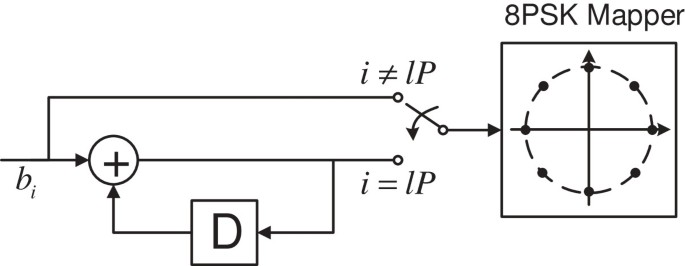Figure 2

## 3 Characteristics of mappings

As mentioned, symbol mapping is crucial for the error performance of BICM-ID. Hence, the analysis of the characteristics of mappings is important. In this section, we use the analysis tools of the EXIT chart and the Euclidean distance spectrum to characterize mappings both intuitionally and theoretically; thus, we obtain a design guideline of irregular mapping optimization. Note that in this section, we only discuss about the characteristics of mappings over AWGN channels. In regard to Rayleigh fading channels, the characteristics of mappings are similar as those for AWGN channels; thus, they are omitted here.

### 3.1 EXIT chart analysis

The EXIT chart is based on mutual information to describe the flow of extrinsic information through the SISO constituent decoders, which is originally proposed in . It is proved to be a powerful analysis tool to provide design guidelines for mappings of an iterative demapping and decoding scheme.

In general, an EXIT chart plots the mutual information I E as a function of I A , where I A is the average a priori information going into the decoder and I E is the average extrinsic information coming out of the decoder. The EXIT chart usually has two EXIT curves for a serially concatenated system, that is, the EXIT curve of the inner decoder and the inverted EXIT curve of the outer decoder. As the EXIT chart is an efficient tool for the convergence prediction of the error performance, the behavior of the decoding algorithm could be approximately predicted before actually running the algorithm, by the following properties [12, 13]:

• If there is a crossing point between the two EXIT curves, the value of this crossing point indicates the error floor of the decoding algorithm.

• If there is an open EXIT tunnel between the two EXIT curves, an infinitesimally low BER could be achieved, when large interleavers and iterations are provided.

• The open tunnel’s area is proportional to how closely can the scheme operate to the channel capacity.

For the BICM-ID with doping system depicted in Figure 1, the two EXIT curves are the EXIT curve of the doped demapper (the inner decoder) and the inverted EXIT curve of the SISO decoder (the outer decoder). This EXIT chart expresses the exchanges of the extrinsic information between these two decoders. In this study, we consider a few typical symbol mappings of 8PSK : the Gray mapping, the set-partitioning (SP) mapping, and the semi-set-partitioning (SSP) mapping. Their EXIT curves are depicted in Figures 3 and 4 at E b /N0=4 dB over AWGN channels, where Figure 3 shows the EXIT curves of the general BICM-ID and Figure 4 shows the EXIT curves of the BICM-ID with doping (doping rate P=50). For reference, we depict the inverted EXIT curve of the SISO decoder simultaneously, and the CC with 2/3 rate and four states  is exploited as the channel encoder.Figure 3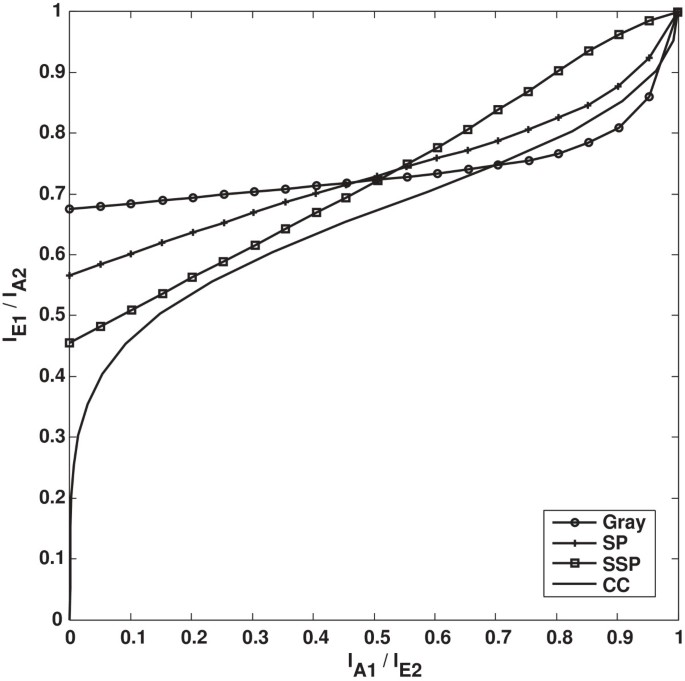Figure 4

The SSP mapping is found to be the best mapping of 8PSK for a general BICM-ID system , which can easily be revealed by the EXIT chart in Figure 3 as well. The EXIT curve of SSP mapping reaches the highest value of $I E 1$, thus yields the lowest error floor. However, even the best mapping of 8PSK cannot create an open EXIT tunnel since the demapper’s EXIT curve cannot reach the point (1,1). As a result, the error performance of the general BICM-ID with 8PSK is limited.

As mentioned, additional doping has a significant influence on mutual information exchanges. With the help of doping, the open EXIT tunnel is conceivable if a proper symbol mapping is provided. As shown in Figure 4, all the three doped demapper’s EXIT curves reach the point (1,1) with P=50. Besides, the SP and SSP curves are both above the inverted SISO decoder’s EXIT curve, that is, open EXIT tunnels are obtained at E b /N0=4 dB over AWGN channels. It is thus clear that better error performances can be achieved with the help of doping.

In order to analyze the influence of doping rate P for the system, we depict the EXIT curves of BICM-ID with SP mapping with doping rate P{0,1,2,10,50,100} over AWGN channels at E b /N0=4 dB, as shown in Figure 5, where the curve with P=0 represents the general BICM-ID without doping. Figure 5 demonstrates that the doping is able to ‘bend up’ the tail of the EXIT curve for high $I A 1$ to reach the point (1,1). However, the small $I A 1$ is lowering with the decrease in doping rate P. According to this property, we obtain that doping rate P can be used to adjust the EXIT curve shape of BICM-ID with a given mapping.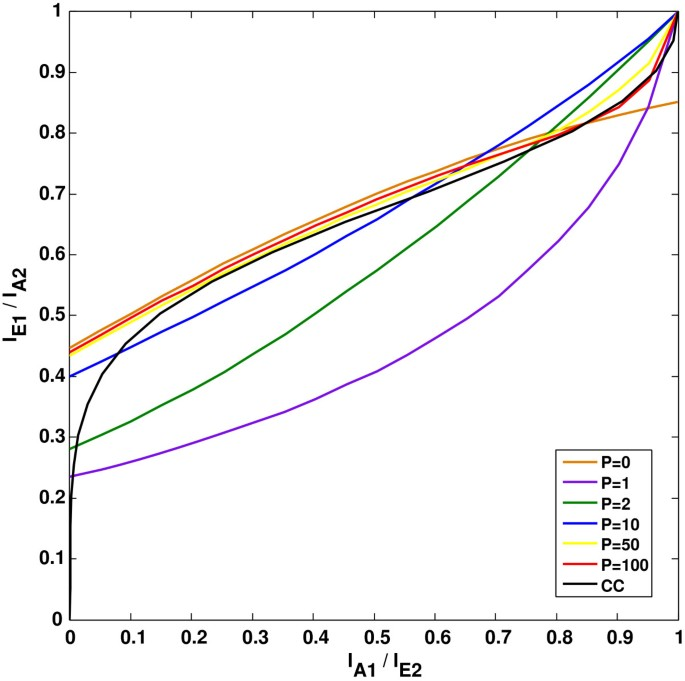Figure 5

### 3.2 Distance spectrum

The Euclidean distance spectrum  is the average or cumulated Hamming distance between bit labels at a specific Euclidean distance, which inherently includes the properties of the signal constellation and mapping. The BSA is designed based on the distance spectrum and is widely used for mapping optimizations of BICM-ID. It searches for the best CF, which shows the influence of the mapping on the pairwise error probability (PEP). The CF is described as 

$D a = 1 m 2 m ∑ i = 1 m ∑ b = 0 1 ∑ s k ∈ χ b i ∑ ŝ k ∈ χ b ̄ i exp − E s 4 N 0 s k − ŝ k 2$
(1)

for AWGN channels, and

$D r = 1 m 2 m ∑ i = 1 m ∑ b = 0 1 ∑ s k ∈ χ b i ∑ ŝ k ∈ χ b ̄ i 1 s k − ŝ k 2$
(2)

for Rayleigh fading channels. χ denotes the signal set of the M-ary PSK constellation, and $χ b i and χ b ̄ i$ denote the two complementary signal subsets, where $b ̄ =1−b∈ 0 , 1$; $s k and ŝ k$ are two coded symbols, where $s k ∈ χ b i$ and $ŝ k ∈ χ b ̄ i$. For an M-ary PSK modulation, m= log2M.

Except for determining corresponding CFs, the Euclidean distance spectrum can also determine the corresponding bit-wise mutual information , thus determining the corresponding EXIT curves. The bit-wise distance spectra are used to classify mappings of the M-ary PSK constellation. A given set of bit-wise distance spectra [W 0 ,W 1 ] characterizes an entire class of equivalent mappings, where W 0 is the bit-wise distance spectrum given no a priori information and W 1 is the bit-wise distance spectrum given full a priori information. W i is expressed as

$W i = w i 1 ( 1 ) w i 1 ( 2 ) ⋯ w i 1 M / 2 ⋮ ⋮ ⋱ ⋮ w i m ( 1 ) w i m ( 2 ) ⋯ w i m M / 2 ,i=0,1.$
(3)
$w 0 j (k)$

denotes the total Hamming distance for the j th bit between one symbol and all other symbols at Euclidean distance d k , averaged over all symbols of the constellation, while $w 1 j (k)$ is denoted similarly for the j th bit when the other bits of a symbol are all known in the demapping process. Therefore, the mappings can easily be classified through an exhaustive search of unique sets of distance spectra [W 0 ,W 1 ].

For 8PSK modulation, the classification reveals 86 different classes of mappings out of a total of 5,040 mappings . Accordingly, the EXIT curves of BICM-ID with 8PSK are classified into 86 classes, which are depicted in Figure 6. Instead of using the exhaustive search for all mappings of 8PSK constellation, 86 unique mappings and 86 unique EXIT curves are now under consideration for the design of our optimization algorithm. Taking advantage of this classification, the computational complexity of the search for suitable mappings is significantly reduced.Figure 6

It is remarkable that the classification is based only on the distance spectra for the two extreme cases of either no a priori or full a priori information; thus, mappings with the same [W 0 ,W 1 ] can still exhibit different distance properties for arbitrary a priori information in between the two extreme cases. Hence, the classification provides a lower bound of the number of unique 8PSK mappings, which leads the optimization algorithm into a suboptimal approach rather than a global optimal one. As a result, there is a trade-off between the complexity and the performance for the design of the optimization algorithm. A significant reduction of the complexity is obtained by the classification, while the near-capacity performance is slightly influenced, which is acceptable for overall consideration.

## 4 Optimization of irregular mapping

To obtain the discrete-input continuous-output memoryless channel (DCMC) capacity associated with 8PSK of 2 bits/channel use, the AWGN channel needs a minimum E b /N0 of 2.75 dB, while the Rayleigh fading channel needs a minimum E b /N0 of 5.37 dB. For a closer approach to the DCMC capacity, the regular modulations and mappings are not effective; thus, the irregular mapping optimization is under consideration. Taking advantages of the characteristics of mappings discussed in Section 3, we investigate a curve-fitting optimization algorithm of finding an EXIT curve of the irregular doped demapper at a minimum E b /N0, while ensuring that this curve is all above the SISO decoder’s inverted EXIT curve and the area within the open EXIT tunnel is as small as possible. Instead of calculating CFs as in the BSA, this optimization is a low-complexity algorithm with the aid of EXIT chart analysis. In addition, the proposed algorithm only focuses on the 86 unique mappings of 8PSK constellation out of a total of 5,040 mappings, which is much more efficient than the exhaustive search algorithms. Furthermore, since the doping rate P can be used to adjust the EXIT curve shape of BICM-ID with a given mapping, a carefully designed irregular doping rate is taken into account for irregular mapping optimization. Note that the optimization algorithm is designed for AWGN and Rayleigh fading channels separately; we have made further efforts to the simultaneous optimization over both AWGN and Rayleigh channels in Section 4.3.

### 4.1 Irregular construction

We divide the codeblock of encoded bits into two subblocks with a coefficient α[0,1]. Two mappings T1,T2{T} are implemented for the two subblocks respectively in every codeblock, as shown in Figure 7, where {T} is the set of the classified unique mappings of 8PSK modulation. Doping rates P1,P2{P} are provided for the two subblocks. A tiny modification of the BICM-ID with doping system is necessary since we only need to alternately select the two mappings and doping rates according to α; the number of constellation points are invariant.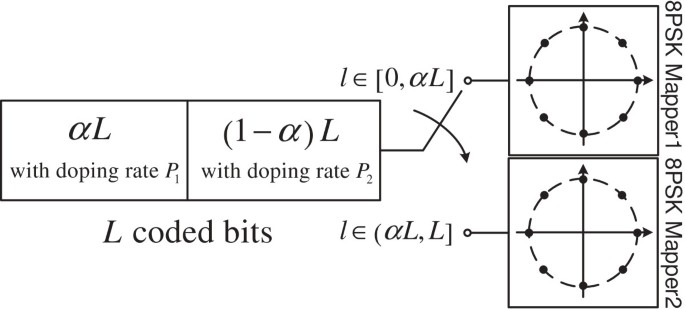Figure 7

It is worth mentioning that doping is able to ‘bend up’ the tail of the curve for high $I A 1$ while lowering the small $I A 1$ with the decreasing doping rate P. On the other hand, dependent values between the adjacent bits are introduced by doping to reduce the error floor; thus, the randomness of the coded bits after interleaving is affected, which will result in performance degradation if the doping rate is too small. As a result, we set the doping rate P{10,50,100} over all considerations.

Denote that $I A 1 P 1 , I E 1 P 1 , I A 2 P 2 , I E 2 P 2 ∈ I A P , I E P$ are the doped demapper’s EXIT curves of mapping T1 with doping rate P1 and mapping T2 with doping rate P2, respectively, where $I A P , I E P$ is the set of the classified unique EXIT curves with doping rate P{10,50,100}. Similarly, denote $I A Ir P Ir , I E Ir P Ir$ as the mutual information of the system with irregular mapping and doping. The irregular mapping is denoted as T I r =[T1,T2], and the corresponding doping rate is P I r =[P1,P2]. Taking advantages of the average approximate of the EXIT chart analysis , we have

$I E 1 P 1 = 1 − 1 L 1 ∑ l 1 = 1 L 1 H b P 1 p l 1 , I E 2 P 2 = 1 − 1 L 2 ∑ l 2 = 1 L 2 H b P 2 p l 2 ,$
(4)

where H b (·) is the binary entropy function and p l is the bit error probability. According to the construction of irregular codeblock shown in Figure 7, $I E Ir P Ir$ is formulated as

$I E Ir P Ir = 1 − 1 L ∑ l = 1 L H b P Ir p l = α 1 − 1 αL ∑ l 1 = 1 αL H b P 1 p l 1 + 1 − α × 1 − 1 1 − α L ∑ l 2 = αL + 1 L H b P 2 p l 2 .$
(5)

From Equations 4 and 5, we arrive at

$I E Ir P Ir =α I E 1 P 1 + 1 − α I E 2 P 2 .$
(6)

Therefore, the doped demapper’s EXIT curve with the irregular mapping T I r =[T1,T2] and doping rate P I r =[P1,P2] is equivalent to the linear combination of the two EXIT curves of mapping T1 with doping rate P1 and mapping T2 with doping rate P2 directly. We obtain that irregular mapping optimization can be transformed to a curve-fitting problem of the classified unique EXIT curves, which calculates the irregular doped demapper’s EXIT curve $I A Ir P Ir , I E Ir P Ir$ at a minimum E b /N0 according to Equation 6, while ensuring that this curve is all above the SISO decoder’s inverted EXIT curve, and the area A I r within the open EXIT tunnel is as small as possible. Hence, the optimal $T Ir opt = T 1 opt , T 2 opt$, $P Ir opt = P 1 opt , P 2 opt$, and α o p t are obtained.

The irregular mapping of the considered two mappings with linear combination of the corresponding EXIT curves can easily be generalized as the condition of N(N>2) mappings, according to :

$I E Ir P Ir = 1 − 1 L ∑ l = 1 L H b P Ir p l = ∑ i = 1 N α i 1 − 1 α i L ∑ l i = 1 α i L H b P i p l i = ∑ i = 1 N α i I E i P i ,$
(7)

where the coefficient α i [0,1] satisfies the condition

$∑ i = 1 N α i =1.$
(8)

It is conceivable that the irregular mapping optimization of N(N>2) mappings may achieve a better error performance than the irregular mapping optimization of two mappings, which is not discussed in this paper and is currently under investigation.

#### Algorithm 1 Irregular mapping optimization### 4.2 Irregular mapping optimization algorithm

The pseudocode of the irregular mapping optimization algorithm is shown in Algorithm 1, where the number of elements in the set of the unique mappings {T} is Q=86, according to the discussion in Section 3.2. The doping rate is Pk{P} with k=1,2,…,K, where we set K=3 according to Section 4.2, that is, {P}={10,50,100}. The EXIT curve of the i th regular mapping with doping rate Pk is denoted as $I A i P i k , I E i P i k ,i=1,2,…,Q$, where $P i k ∈{P}$ with k=1,2,…,K. The SISO decoder’s inverted EXIT curve is denoted as $I A CC , I E CC$.

For the sake of achieving a near-capacity performance, the minimum E b /N0 will be determined before searching for the optimal irregular mapping. We set an experience factor δ=(δ1,δ2,δ3),δ1>δ2>δ3>0 to adjust the initial value and the searching step size of E b /N0. First, E b /N0 is initialized as E b /N0=C l i m +δ1, where C l i m is the DCMC capacity limit valued at 2.75 dB for AWGN channels and 5.37 dB for Rayleigh fading channels. δ1 is large enough to ensure that at least one open EXIT tunnel is existing at the initial E b /N0 between the demapper’s EXIT curve of the regular mapping $I A P , I E P$ and the SISO decoder’s inverted EXIT curve. Second, the value of E b /N0 is slightly decreasing with δ2 until no open EXIT tunnel is obtained with the regular mappings. Then, the searching for the curve $I A Ir P Ir , I E Ir P Ir$ of the optimal irregular mapping is activated, the optimization algorithm will find a minimum E b /N0 while ensuring that the EXIT curve is all above the SISO decoder’s inverted EXIT curve, and the area A I r within the open EXIT tunnel is as small as possible. If no irregular mapping is found, E b /N0 will slightly increase with δ3. In this study, the experience factor is set as δ=(δ1,δ2,δ3)=(0.5,0.05,0.01), according to the practical experiments.

The minimum E b /N0 obtained by Algorithm 1 is E b /N0=3 dB over AWGN channel. Figure 8 demonstrates the upper and lower bounds of the doped demapper’s EXIT curves with doping rate P{10,50,100} at E b /N0=3 dB over AWGN channels, by calculating all the 86 unique doped demapper’s EXIT curves of 8PSK mappings. It is shown that there is still an open tunnel between the upper bound of the doped demapper’s EXIT curve and the SISO decoder’s inverted EXIT curve; thus, the optimal irregular mapping is conceivable.Figure 8

The EXIT curve of the optimal irregular mapping over AWGN channels is depicted in Figure 9, where E b /N0=3 dB is the minimum E b /N0 to create an open EXIT tunnel. The gap between the minimum E b /N0 and the DCMC capacity limit is 0.25 dB. The optimal irregular mapping $T Ir opt a = T 1 opt a , T 2 opt a$ is obtained with $P Ir opt a = 50 , 50$ and $α opt a =0.4$.Figure 9

Similarly, irregular mapping optimization is implemented over Rayleigh fading channels (in this study, we focus on the independent identically distributed Rayleigh fading channels); all the other conditions are the same as those for AWGN channels. The upper and lower bounds over Rayleigh fading channels at the minimum E b /N0=5.7 dB is depicted in Figure 10. Figure 11 shows the EXIT curve of the optimal irregular mapping. The gap between the minimum E b /N0 and the DCMC capacity limit is 0.33 dB. The optimal irregular mapping $T Ir opt r = T 1 opt r , T 2 opt r$ is obtained with $P Ir opt r = 50 , 100$ and $α opt r =0.5$.Figure 10Figure 11

### 4.3 Optimization trade-off between AWGN and Rayleigh fading channels

The proposed optimization algorithm in Section 4.2 is designed for AWGN and Rayleigh fading channels separately; thus, the optimal irregular mapping suited for AWGN channels can obviously not achieve the optimal performance over Rayleigh fading channels. As a result, the proposed optimization algorithm is infeasible for the case of a simultaneous optimization over both AWGN and Rayleigh fading channels. The technique of SSD [10, 18, 19] provides a significant diversity gain over Rayleigh fading channels while it leads to nondegradation over AWGN channels, which is suitable for the case of the simultaneous optimization. With the aid of SSD, we give a preliminary investigation of the optimization trade-off between AWGN and Rayleigh fading channels.

For transmission over Rayleigh fading channels, the module of SSD is cascaded to the proposed BICM-ID with doping scheme, which is depicted in Figure 12. The diversity order is improved by optimally rotating the constellation and separately interleaving the coordinates. We make use of the design criterion of obtaining an optimal rotation for the proposed system in Figure 12, which is expressed asFigure 12
$D= 1 m 2 m ∑ i = 1 m ∑ b = 0 1 ∑ s k ∈ χ b i ( θ ) f s k − 1 ,$
(9)

where $f s k = max ŝ k ∈ χ b ̄ i ( θ ) 1 Re s k − ŝ k 2 + 1 Im s k − ŝ k 2$. The symbolic representations in Equation 9 are the same as those of the CF for Rayleigh fading channels in Equation 2. The difference between Equations 9 and 2 is that value D in Equation 9 depends on the rotation angle θ, and the optimal θ is chosen by maximizing D.

Since we have obtained the optimal $T Ir opt a$, $P Ir opt r$, and $α opt a$ over AWGN channels in Section 4.2, we use exactly the same parameters for the simultaneous optimization between AWGN and Rayleigh fading channels, in order to firstly maintain the near-capacity performance over AWGN channels. Then, the optimal parameters for AWGN channel is used for the BICM-ID-SSD with doping scheme over Rayleigh fading channels. With the aid of SSD, the design criterion in Equation 9 is used for choosing an optimal rotation angle θ; thus, a better performance is achieved over Rayleigh fading channels. For the reason that the SSD has no influence on transmissions over AWGN channels, the proposed BICM-ID-SSD with doping scheme with the optimal rotation angle θ will achieve a near-capacity performance for both AWGN and Rayleigh fading channels.

We obtain the optimal rotation angle θ=9° for the proposed BICM-ID-SSD with doping for 8PSK with the 2/3-rate, four-state CC, according to the optimization criterion in Equation 9. The optimal rotation angle is the same as that in  for BICM with 8PSK and 2/3-rate CC. The EXIT curve of the proposed BICM-ID-SSD with irregular mapping and doping over Rayleigh fading channels is depicted in Figure 13, where the parameters of irregular mapping and doping are exactly the same as the optimal parameters for AWGN channels. For comparison, we also depict the EXIT curve without constellation rotation.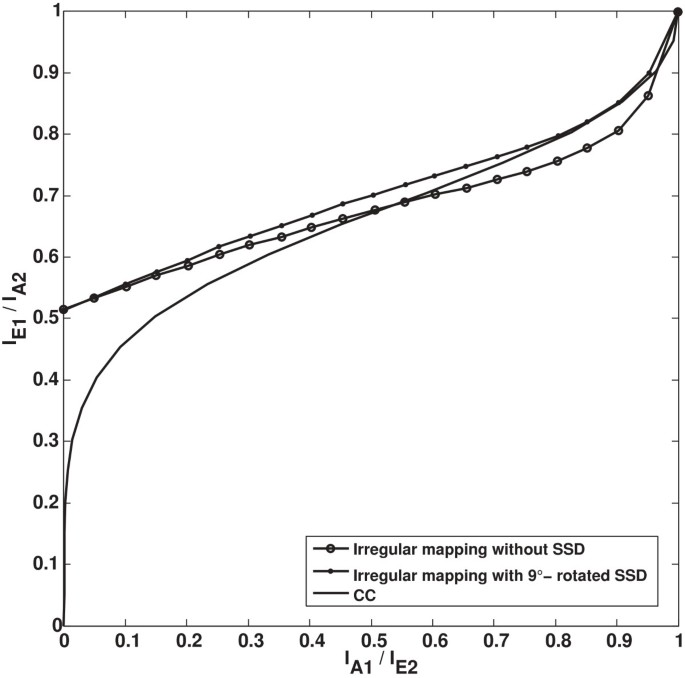Figure 13

As shown in Figure 13, the demapper’s EXIT curve with the optimal rotation angle θ=9° becomes steeper than the curve without rotation, which is the same as mentioned in . With the aid of SSD, the open EXIT tunnel between the demapper’s EXIT curve and the SISO decoder’s inverted EXIT curve is obtained over Rayleigh fading channels, while using the optimal $T Ir opt a$, $P Ir opt r$, and $α opt a$ over AWGN channels. Since no performance degradation is caused by SSD for transmissions over AWGN channels, the proposed BICM-ID-SSD with doping for 8PSK with the rotation angle θ=9° performs the same as the separate optimization over AWGN channels. In other words, the simultaneous optimization over both AWGN and Rayleigh fading channels reaches the same performance as the separate optimization algorithm over AWGN and Rayleigh fading channels. However, the optimization criterion is based on the harmonic mean of the square of Euclidean distance , which is not effective enough as mentioned in . Further study is needed for an effective optimization, which is now under investigation.

## 5 Simulation results

This section provides the simulation results to confirm the advantages of the proposed optimal irregular mappings of 8PSK with BICM-ID with irregular doping. We also provide the simulation of the general BICM-ID for comparison. The 2/3-rate, four-state CC is implemented as the encoder, and the generator sequences are g 1 =(6,2,6), g 2 =(2,4,4) . The spectral efficiency is 2 bits/channel use, which yields a DCMC capacity limit of 2.75 dB for AWGN channels and 5.37 dB for Rayleigh fading channel. We set the block length of the proposed system to 200,000 bits and the iterations to 50.

The BER performances of BICM-ID systems are presented in Figure 14 over AWGN channels and in Figure 15 over Rayleigh fading channels, respectively, including the performances of both the general BICM-ID system and the BICM-ID with doping system. For the general BICM-ID system, the typical symbol mappings  (Gray, SP, and SSP) are employed. For the BICM-ID with doping system, we employ typical symbol mappings with the doping rate P=50 and optimal irregular mappings with irregular dopings, which are obtained in Section 4. For AWGN channels, it is shown in Figure 14 that the curve of BICM-ID using the optimal irregular mapping and doping outperforms the other mappings. It yields a gain of about 0.1 dB at the BER of 10−4 than the SP mapping of BICM-ID with doping, which is the best out of the other BER curves with typical mappings. While for Rayleigh fading channels, Figure 15 demonstrates that the BER curve of BICM-ID with the optimal irregular mapping and doping also performs best and yields a gain of about 0.5 dB at the BER of 10−4 than the second best BER curve. As expected, the doping module allows to reduce the theoretical error floor to a value too low to be observed in the simulations both in Figures 14 and 15.Figure 14Figure 15

The gap between the DCMC capacity limit and the optimal curve at BER= 10−4 is 0.5 dB for AWGN channels and 0.73 dB for Rayleigh fading channels, that is, the maximum achievable capacity of the proposed optimal irregular mapping is 3.25 dB over AWGN channels and 6.1 dB over Rayleigh channels. The decoding trajectories of the BICM-ID with the proposed optimal irregular mappings are depicted in Figures 16 and 17, at E b /N0=3.25 dB for AWGN channels and E b /N0=6.1 dB for Rayleigh fading channels, respectively. The trajectories are well matched to the corresponding EXIT curves, and the iterations needed for reaching the point (1,1) are less than 30 over both AWGN and Rayleigh channels. However, it is worth mentioning that the BER performance of BICM-ID with the proposed optimal irregular mapping and doping is slightly worse than the suggestion of the EXIT chart analysis in Section 4 for both AWGN and Rayleigh fading channels. The reason is that the BER performance is restricted to the size of the interleaver and the iterations. A potential improvement of the BER performance is predictable due to the implementation of an ideal interleaver and iterations regardless of the high complexity.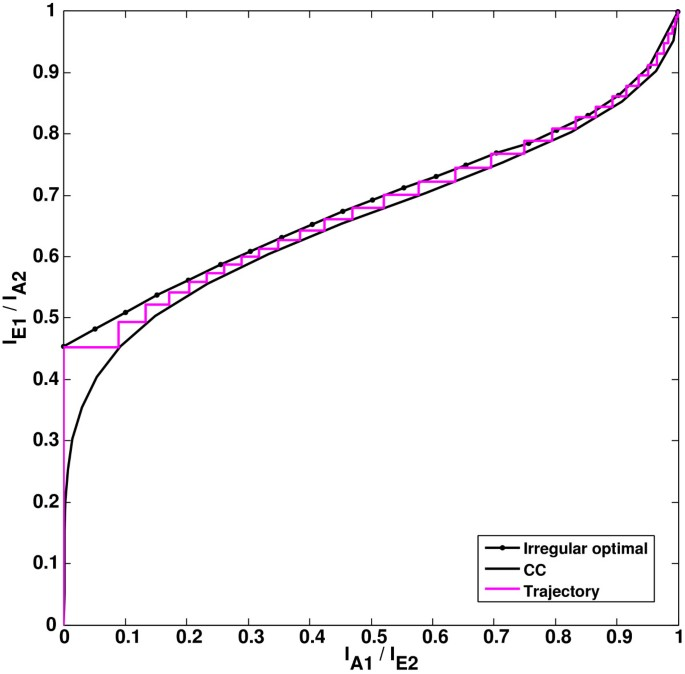Figure 16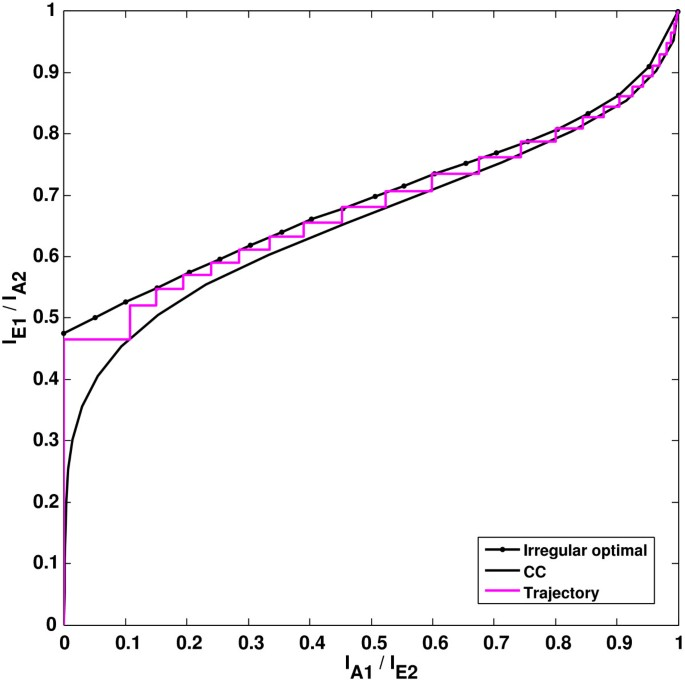Figure 17

## 6 Conclusions

In this paper, we have proposed a low-complexity irregular mapping optimization for BICM-ID with irregular doping and 8PSK. The Euclidean distance spectrum and the EXIT chart are aided for the proposed optimization to provide design guidelines of mappings, and the computational complexity is largely decreased by the classification of mappings. The simulation results demonstrate that the proposed optimal irregular mapping and doping yield a better performance than the typical symbol mappings of 8PSK, that is, a 0.1-dB gain over AWGN channels and a 0.5-dB gain over Rayleigh fading channels at BER= 10−4. In addition, the optimal irregular mapping performs about 0.5 and 0.73 dB away from the DCMC capacity limit over AWGN and Rayleigh channels, respectively, at BER= 10−4 and for the spectral efficiency of 2 bits/channel use.

## References

1. 1.

Zehavi E: 8-PSK trellis codes for a Rayleigh channel. IEEE Trans. Commun 1992, 40(5):873-884. 10.1109/26.141453

2. 2.

Caire G, Taricco G, Biglieri E: Bit-interleaved coded modulation. IEEE Trans. Inf. Theory 1998, 44(3):927-946. 10.1109/18.669123

3. 3.

Li X, Ritcey J: Bit-interleaved coded modulation with iterative decoding using soft feedback. Electron. Lett 1998, 34(10):942-943. 10.1049/el:19980651

4. 4.

ten Brink S, Speidel J, Yan RH: Iterative demapping and decoding for multilevel modulation. In IEEE Global Telecommunications Conference, 1998. GLOBECOM 1998. The Bridge to Global Integration. Piscataway: IEEE; 1998:579-584.

5. 5.

Li X, Chindapol A, Ritcey JA: Bit-interleaved coded modulation with iterative decoding and 8 PSK signaling. IEEE Trans. Commun 2002, 50(8):1250-1257. 10.1109/TCOMM.2002.801524

6. 6.

Pfletschinger S, Sanzi F: Error floor removal for bit-interleaved coded modulation with iterative detection. IEEE Trans. Wireless Commun 2006, 5(11):3174-3181.

7. 7.

Schreckenbach F, Bauch G: Bit-interleaved coded irregular modulation. Eur. Trans. Telecommunications 2006, 17(2):269-282. 10.1002/ett.1107

8. 8.

Tee R, Maunder RG, Hanzo L: Exit-chart aided near-capacity irregular bit-interleaved coded modulation design. IEEE Trans. Wireless Commun 2009, 8: 32-37.

9. 9.

Schreckenbach F, Gortz N, Hagenauer J, Bauch G: Optimized symbol mappings for bit-interleaved coded modulation with iterative decoding. In IEEE Global Telecommunications Conference, 2003 (GLOBECOM’03). Piscataway: IEEE; 2003:3316-3320.

10. 10.

Xie Q, Yang Z, Song J, Hanzo L: EXIT-chart-matching-aided near-capacity coded modulation design and a BICM-ID design example for both Gaussian and Rayleigh channels. IEEE Trans. Vehicular Technol 2013, 62(3):1216-1227.

11. 11.

ten Brink S: Convergence behavior of iteratively decoded parallel concatenated codes. IEEE Trans. Commun 2001, 49(10):1727-1737. 10.1109/26.957394

12. 12.

Ashikhmin A, Kramer G, ten Brink S: Extrinsic information transfer functions: model and erasure channel properties. IEEE Trans. Inf. Theory 2004, 50(11):2657-2673. 10.1109/TIT.2004.836693

13. 13.

Hagenauer J: The EXIT chart-introduction to extrinsic information transfer in iterative processing. Proceedings of the 12th European Signal Processing Conference (EUSIPCO), Vienna, 6–10 Sept 2004 1541-1548.

14. 14.

Brannstrom F, Rasmussen LK: Classification of unique mappings for 8PSK based on bit-wise distance spectra. IEEE Trans. Inf. Theory 2009, 55(3):1131-1145.

15. 15.

Schreckenbach F, Bauch G: Irregular signal constellations, mappings and precoder. International Symposium on Information Theory and its Applications (ISITA), Parma, 10–13 Oct 2004 1332-1336.

16. 16.

Szczecinski L, Chafnaji H, Hermosilla C: Modulation doping for iterative demapping of bit-interleaved coded modulation. Commun. Lett. IEEE 2005, 9(12):1031-1033. 10.1109/LCOMM.2005.1576578

17. 17.

Liu Z, Peng K, Cheng T, Wang Z: Irregular mapping and its application in bit-interleaved LDPC coded modulation with iterative demapping and decoding. IEEE Trans. Broadcasting 2011, 57(3):707-712.

18. 18.

Chindapol A, Ritcey JA: Bit-interleaved coded modulation with signal space diversity in Rayleigh fading. In Conference Record of the Thirty-Third Asilomar Conference on Signals, Systems, and Computers 1999. Piscataway: IEEE; 1999:1003-1007.

19. 19.

Xie Q, Song J, Peng K, Yang F, Wang Z: Coded modulation with signal space diversity. IEEE Trans. Wireless Commun 2011, 10(2):660-669.

20. 20.

Lin S, Costello DJ: Encoding of convolutional codes. In Error Control Coding. Englewood Cliffs: Prentice-Hall; 2004:287-295.

Download references

## Acknowledgements

This research work was supported by the State Key Program of National Natural Science Foundation of China (grant no. 61032006), the National Science Foundation of China (grant no. 61271282), and the Award Foundation of Chinese Academy of Sciences (grant no. 2069901).

## Author information

Correspondence to Chen Cheng or Guofang Tu.

## Additional information

### Competing interests

The authors declare that they have no competing interests.

## Authors’ original submitted files for images

Below are the links to the authors’ original submitted files for images.

## Rights and permissions

Open Access This article is distributed under the terms of the Creative Commons Attribution 2.0 International License (https://creativecommons.org/licenses/by/2.0), which permits unrestricted use, distribution, and reproduction in any medium, provided the original work is properly cited.

Reprints and Permissions

## About this article

• #### DOI

https://doi.org/10.1186/1687-1499-2014-31

### Keywords

• Bit-interleaved coded modulation with iterative decoding (BICM-ID)
• Irregular mapping
• Doping
• Distance spectrum
• Extrinsic information transfer (EXIT) chart